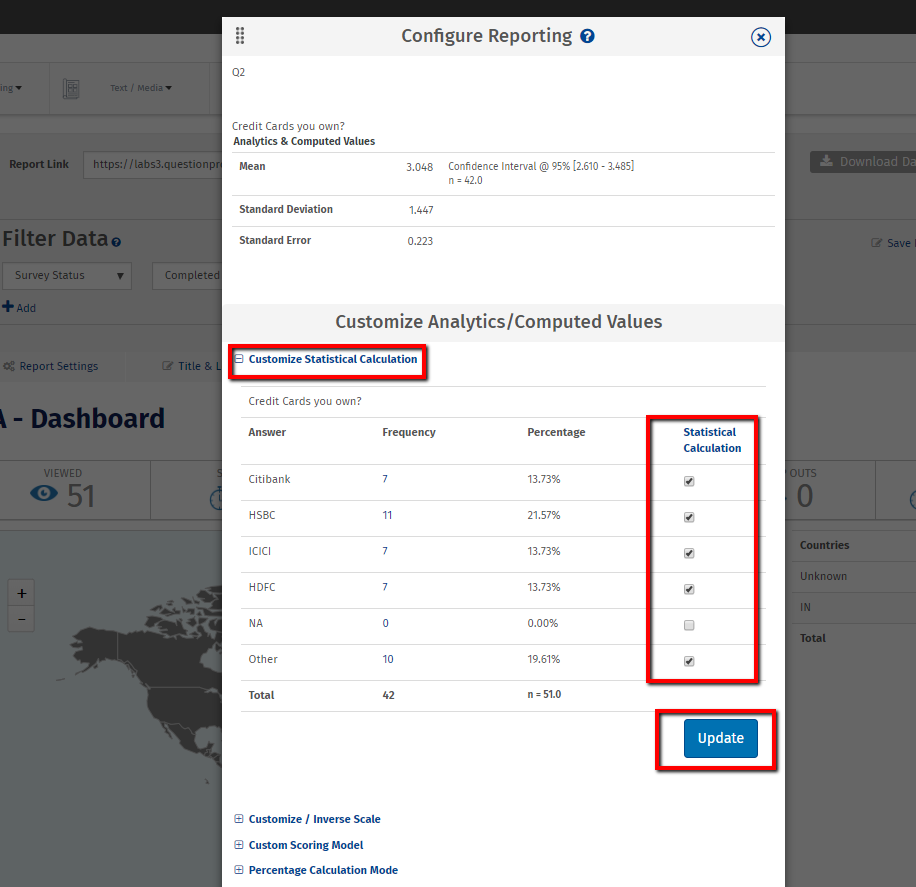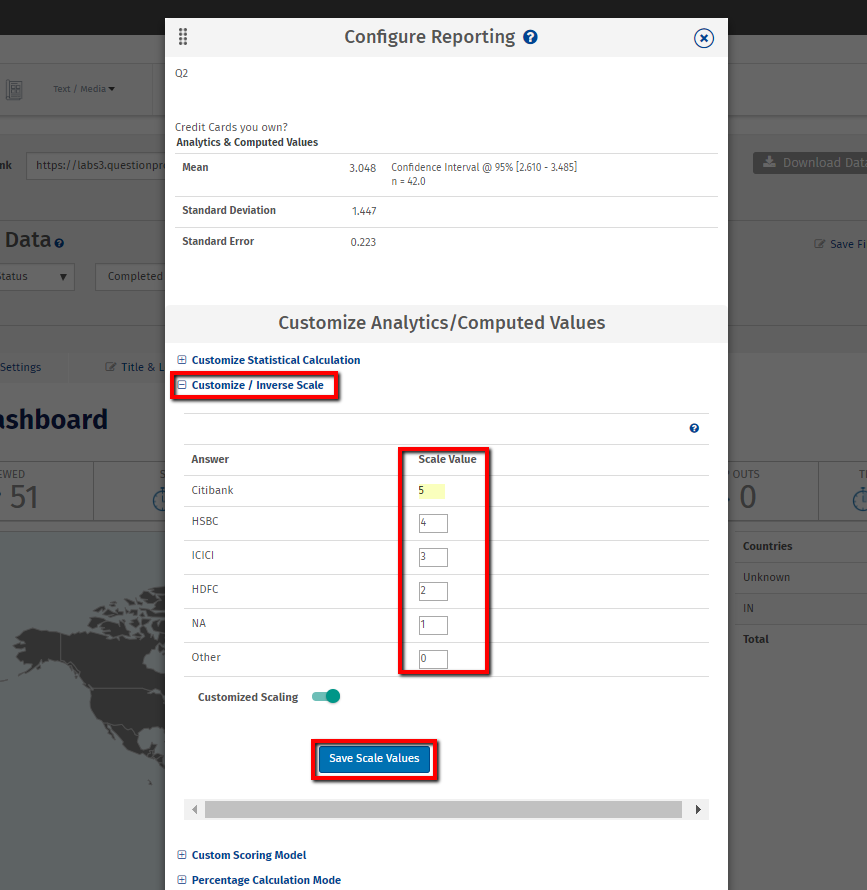What is weighted mean?

Calculating the mean:

By default, for any question, the first answer option is given a score of 1, the second answer option a score of 2, etc. The formula used to calculate a mean is as follows. Xn = number of responses (such as 3 responses for the first answer option, 5 responses for the second answer option, etc.) Wn = score (default of 1 for the first answer option, 2 for the second answer option, etc.) n = total number of responses obtained for the question MEAN = [(X1 * W1)+ (X2 * W2) + (X3* W3) +… (Xn * Wn)]/n

How can I customize the Statistical Calculations?

To customize the Statistical Calculations, Go to:

• Login »  Surveys »  Reports »  Dashboard
•• Click the gear icon next to the question for which you want to customize the Statistical Calculations.
• Click Customize Statistical Calculation: uncheck the boxes next to the answer options you want to exclude from all calculations,data table,reports and chart.
• Click Update.
• The new calculations can be found in the same location as before, under the answer options table.

In my case, the first option does not necessarily represent 1 — in fact, the scores for my scales are reversed. How can I adjust the scores to be reflected in the mean?

To adjust scores, you’ll want to use the Custom/Inverse Scale.

• Click the gear icon next to the question for which you want to customize the mean.
• Click Custom/Inverse Scale: next to each answer option, enter the weight you want to assign to the answers, then check the box next to Customized Scaling to show the customized scale mean.
• Click Save Scale ValuesI have a side-by-side matrix in my survey. What is the Weighted Average?

The weighted average scoring model applies a “weight” to the matrix questions based on responses to the first item in a side-by-side matrix. Generally the first item measures attributes like “Importance,” “Need,” or “Expectation”. In such cases, the values of the subsequent dimensions (“Satisfaction” , “Use” or “Delivery” etc.) can weighed based on corresponding scores from the first attribute.

So how is the weight for each item calculated?

For each Side-By-Side matrix question, there is an “Anchor” dimension. This is the first dimension listed, and we call it the anchor. In most cases, this is the “Importance” or “Expectation” dimension. NOTE – THIS HAS TO BE THE FIRST DIMENSION YOU MEASURE. QuestionPro automatically assumes that the first dimension is the anchor. All subsequent calculations are based on the anchor. The weight for each item in the anchor is calculated by this formula: Weight(n) = Anchor Score(n) / Sum of [Anchor Score]

How is the Adjusted Score for the other dimensions calculated?

Adjusted Score(n) = Weight(n) * Score(n)